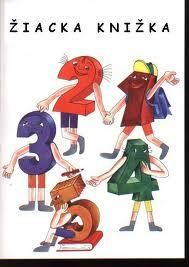# Pupils 5753

The class has 24 pupils, with 40% fewer boys than girls. How many boys and girls are in the class?

c =  9
d =  15

### Step-by-step explanation:

c+d=24
c = d - 40/100 d

c+d=24
c = d - 40/100•d

c+d = 24
100c-60d = 0

c = 9
d = 15

Our linear equations calculator calculates it.Did you find an error or inaccuracy? Feel free to write us. Thank you!

Tips for related online calculators